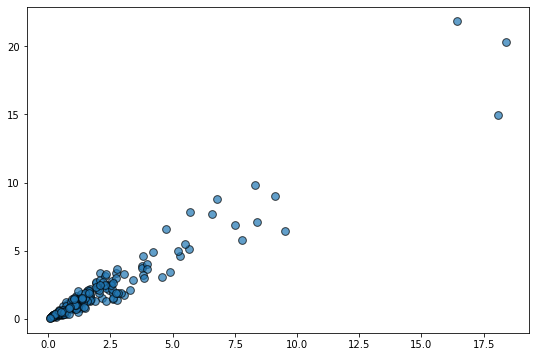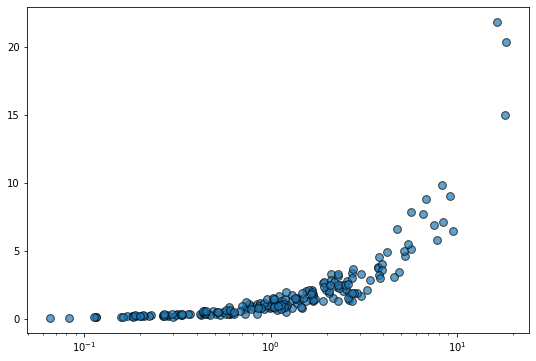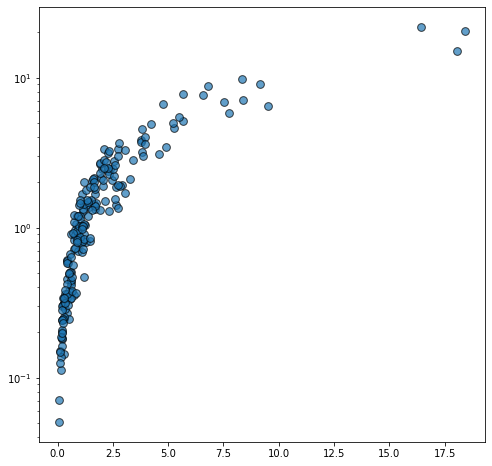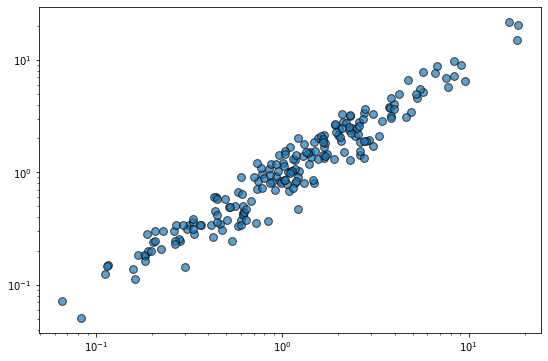# Scatterplot and log scale in Matplotlib

This guide shows how to create a scatterplot with log-transformed axes in Matplotlib. This post uses the object oriented interface and thus uses `ax.set_xscale('log')`, but this can also be achieved with `plt.xscale('log')` if you're using `plt.plot()`

Let's get started by importing Matplotlib and Numpy

``````import matplotlib.pyplot as plt
import numpy as np``````

Let's get started by creating a reproducible random number generator. This ensures the result is the same no matter how many times we generate the random data.

``rng = np.random.default_rng(1234)``

The next step is to generate some random data where it makes sense to apply a logarithmic transformation to make it easier to see the relationship between the variables.

In this case, we're going to generate data that violates the homoscedasticity assumption of ordinary linear regression. This is just statistical jargon that means that the variability of the y variable is not constant for all the values of x. In this case, the variability of y increases as the value of x increases.

``````# Generate data
x = rng.lognormal(size=200)
y = x + rng.normal(scale=5 * (x / np.max(x)), size=200)

# Initialize layout
fig, ax = plt.subplots(figsize = (9, 6))

ax.scatter(x, y, s=60, alpha=0.7, edgecolors="k");``````Let's say the horizontal scale is logarithmic now:

``````fig, ax = plt.subplots(figsize = (9, 6))
ax.scatter(x, y, s=60, alpha=0.7, edgecolors="k")

# Set logarithmic scale on the x variable
ax.set_xscale("log");``````And what if the vertical scale is logarithmic?

``````fig, ax = plt.subplots(figsize = (8,8))
ax.scatter(x, y, s=60, alpha=0.7, edgecolors="k")

# Set logarithmic scale on the y variable
ax.set_yscale("log");``````Let's use a logarithmic scale for both axes now:

``````fig, ax = plt.subplots(figsize = (9, 6))
ax.scatter(x, y, s=60, alpha=0.7, edgecolors="k")

# Set logarithmic scale on the both variables
ax.set_xscale("log")
ax.set_yscale("log");``````The relationship between the variables is linear in this log-transformed space and the variability of y looks constant. So cool!

## Contact & Edit

👋 This document is a work by Yan Holtz. Any feedback is highly encouraged. You can fill an issue on Github, drop me a message onTwitter, or send an email pasting `yan.holtz.data` with `gmail.com`.